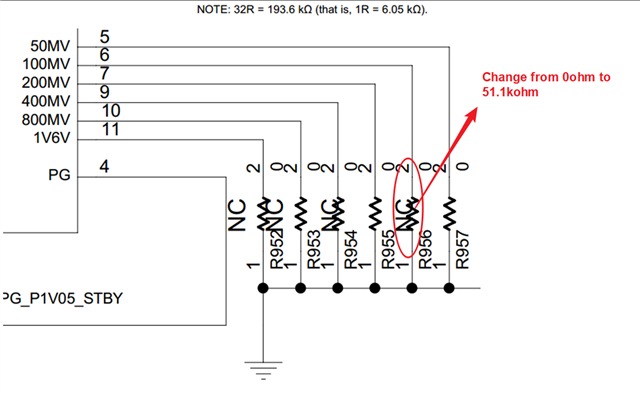If you have a related question, please click the "Ask a related question" button in the top right corner. The newly created question will be automatically linked to this question.

# TPS7A8300: Programmable output voltage function consult

Part Number: TPS7A8300

Hi Team,

Customer wants to adjust their output voltage by 10~20mV, so the minimum resolution 50mA is too large for them. So they want to change the R956 resistor from 0ohm to 51.1kohm for getting smaller voltage adding.

Could you pls help review the potential risk for above scenario? Is that okay to do that?Best,

Stanley

• Hi Stanley,

I am not sure what the target voltage values of the customer are, but in my opinion perhaps a better way would be to reduce the 50mV increment (obtained by using R957) to a 12.5mV increment (since this is in the desired resolution range). The functional block diagram (Section 7.2, Page 17 of the Datasheet) shows that the value of RFB_BOT for 50mV is 32*R (where R = 6.05KΩ). If this value was 128*R instead, then this pin would provide 12.5mV increment. Therefore if R957 was replaced with a resistor equal to 96*R (since 96*R + 32*R = 128*R and 96*R ~= 576KΩ) then the increment in voltage would be 12.5mV. For example, in the image that you have attached, if R957 was replaced with a 576K resistor and only R956 was left in place (R952, R953, R954 & R955 removed) then the net output voltage would be 800mV + 100mV + 12.5V = 912.5mV. Using a 51.1K resistor would perhaps provide a desired target voltage for that pin, but the additive property would be lost.

Another alternative method to reduce resolution, but at the cost of range, is described in Section 9.1.1.4 of the following Datasheet. One could also use the FB node and and custom feedback resistors to generate desired output voltage. Hope this answered your query. Thanks!

Regards,

Srikanth